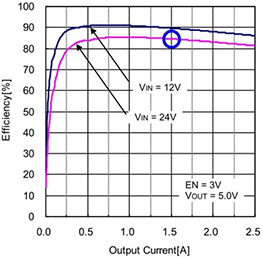Technical Information Site of Power Supply Design

2019.01.10 DC/DC

# Simplified Method of Loss Calculation

Examination of Losses

In the previous article, we presented the method for calculating total losses for a power supply IC by determining and adding the losses for each part. This time, we describe a method, which is however somewhat simplified, for determining the losses of a power supply IC using existing data.

Simplified Method of Loss Calculation

In many cases, the data sheet of a power supply IC includes a graph of efficiency measured for a standard application circuit (efficiency versus output current). If the circuit conditions used and the efficiency curve conditions are the same or are similar, then it can be assumed that more or less the same efficiency curve will be obtained for a circuit you have designed yourself. Using this, it is possible to calculate losses in a simplified manner. Similarly to the calculation example of the previous article, we use an example of a synchronous rectifying step-down converter with an internal MOSFET.

To begin with, and in order to review the relationship between efficiency and losses, we present the equation for calculating losses from the efficiency.

Input power [W]＝Output power[W]＋Loss[W]
Efficiency（×100 for a percent representation）＝ Output power [W]÷ Input power [W]

Loss [W] ＝ Output power [W] ×（1－efficiency）÷ efficiency

We use the following conditions to calculate using the efficiency curve.

Conditions of use: Vin＝24 V, Vout＝5 V, Iout＝1.5 A

From the graph, the efficiency is 84%　（blue circle）

Loss [W]＝Output power [W]×（1－Efficiency）÷Efficiency
＝（5V×1.5A）×（1－0.84）÷0.84＝1.43WThe loss calculated here is the loss for the circuit (similarly to the efficiency), and so, similarly to the previous calculation example, the conduction loss (PCOIL) due to the DCR of the external output inductor must be subtracted. For information on inductor losses, refer to this explanation.

In this way, an approximation of the loss can be calculated from the efficiency curve. We just stated that the loss of the external inductor is omitted, but stated somewhat more precisely, the approximate value includes losses due to other external components, the thin film wiring on a PCB, and the like. However, the loss for the power supply IC itself is smaller than this value (normally only slightly smaller), and so there is no particular problem in using the value as an estimate.

When an external power transistor is used, an estimate can be made using the same approach, but in any case the losses of the power transistor must be determined separately, and so the trouble involved may not be very different from performing separate calculations.

Finally, fractions resulting from calculations should as a rule be rounded up rather than rounded down. The number of digits to use should be decided based on the number of decimals that are valid (relative to the overall power). This is done in order to opt for safety in dealing with errors, and proper care should be taken with respect to negative factors such as losses and heat generation. Of course an operating margin is desirable when deciding whether a component can be used.

#### Key Points:

・When the data sheet for a power supply IC provides an efficiency curve, the loss can be calculated from the efficiency by a simple procedure.

This website uses cookies.

By continuing to browse this website without changing your web-browser cookie settings, you are agreeing to our use of cookies.